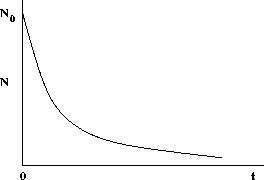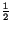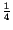# Half life

The half-life of a radioactive substance is a measure of how quickly radioactive decay takes place. Suppose at some initial time we start with N0 radioactive nuclei, and then measure the number N at a later time t. One finds N behaves as in the following graph.It is convenient to introduce the half-life, T1/2, of a substance, defined as the time after which exactly one half of a the originally active nuclei remain. In terms of this, the number of nuclei N remaining at time t, given N0 at time t = 0, is given byThus, after a period of one half-life,of a substance remains, after another half lifex=of a substance remains, and so on. Half-lives of substances range from tiny fractions of a second to millions of years.

modtech@theory.uwinnipeg.ca
1999-09-29